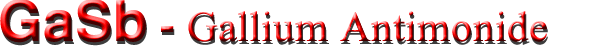Band structure and carrier concentration

Basic Parameters
Temperature Dependences
Dependence of the Energy Gap on Hydrostatic Pressure
Energy Gap Narrowing at High Doping Levels
Effective Masses
Donors and Acceptors

Basic Parameters

 Energy gap 0.726 eV Energy separation (EΓL) between Γ and L valleys 0.084 eV Energy separation (EΓX) between Γ and X valleys 0.31 eV Energy spin-orbital splitting 0.80 eV Intrinsic carrier concentration 1.5·1012 cm-3 Intrinsic resistivity 103 Ω·cm Effective conduction band density of states 2.1·1017 cm-3 Effective valence band density of states 1.8·1019 cm-3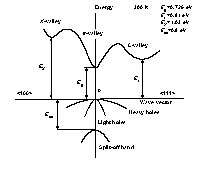Band structure and carrier concentration of GaSb. 300 K Eg= 0.726 eV EL = 0.81 eV EX = 1.03 eV Eso = 0.8 eV

Temperature Dependences

Temperature dependence of the energy gap(Wu and Chen )

Eg = 0.813 - 3.78·10-4·T2/(T+94) (eV),
where T is temperature in degrees K (0 < T < 300).

Temperature dependence of energy EL

EL = 0.902 - 3.97·10-4·T2/(T+94) (eV)

Temperature dependence of energy EX(Lee and Woolley )

EX = 1.142 - 4.75·10-4·T2/(T+94) (eV)

Effective density of states in the conduction band

Nc = 4.0·1013·T3/2 (cm-3)

Effective density of states in the conduction band

Nc = 4.0·1013·T3/2 (cm-3)

Effective density of states in the valence band

Nv = 3.5·1015·T3/2 (cm-3)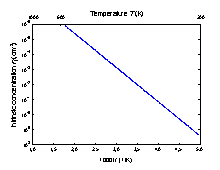The temperature dependences of the intrinsic carrier concentration.

Dependences on Hydrostatic Pressure

Eg = Eg(0) + 14.5·10-3P (eV)
EL = EL(0) + 5.0·10-3P (eV)
EX = EX(0) - 1.5·10-3P (eV),
where P is pressure in kbar.

Energy Gap Narrowing at High Doping Levels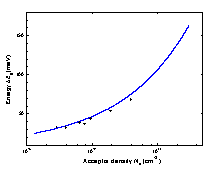Energy gap narrowing versus acceptor acceptor doping density. Curve is calculated for p-GaSb according to Jain et al. . Points show experimental results (Titkov et al. ).

For n-type GaSb(Jain et al. ):

Eg = 13.6·10-9·Nd1/3 + 1.66·10-7·Nd1/4 + 119·10-12·Nd1/2 (eV)

For p-type GaSb(Jain et al. ):

Eg = 8.07·10-9·Na1/3 + 2.80·10-7·Na1/4+ 4.12·10-12·Na1/2 (eV)

Effective Masses

Electrons:

 For Γ-valley mΓ = 0.041mo In the L- valley the surfaces of equal energy are ellipsoids ml= 0.95mo mt= 0.11mo Effective mass of density of states mL= 16(mlmt2)1/3= 0.57mo In the X- valley the surfaces of equal energy are ellipsoids ml= 1.51mo mt= 0.22mo Effective mass of density of states mX= 9(mlmt2)1/3= 0.87mo

Holes:

 Heavy mh = 0.4mo Light mlp = 0.05mo Split-off band mso = 0.14mo Effective mass of density of states mv = 0.8mo Effective mass of density of conductivity (Heller and Hamerly ) mvc = 0.3mo

Donors and Acceptors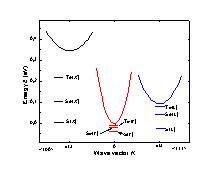The diagram of IV group donor states (Vul' et al. ).

Ionization energies of shallow donors (eV)

 Te(L) Te(X) Se(L) Se(X) S(L) S(X) ~0.02 ≤0.08 ~0.05 ~0.23 ~0.15 ~0.30
For typical donor concentrations Nd≥ 1017 cm-3 the shallow donor states connected with Γ-valley did not appear.

Ionization energies of shallow acceptors (eV):

The dominant acceptor of undoped GaSb seems to be a native defect.
This acceptor is doubly ionizable
 Ea1 Ea2 Si Ge Zn 0.03 0.1 ~0.01 ~0.009 ~0.037